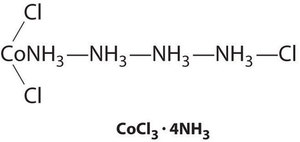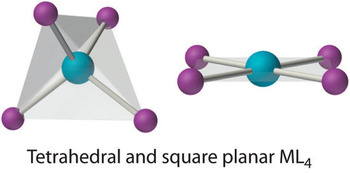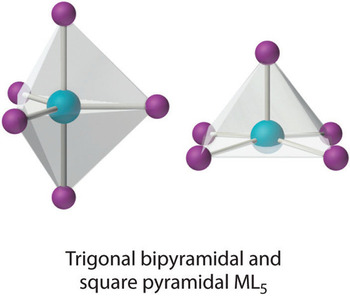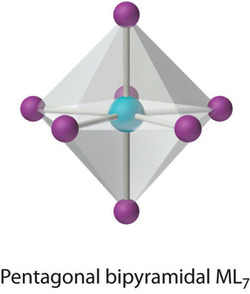# 20.1: Werner’s Theory of Coordination Compounds

$$\newcommand{\vecs}{\overset { \rightharpoonup} {\mathbf{#1}} }$$ $$\newcommand{\vecd}{\overset{-\!-\!\rightharpoonup}{\vphantom{a}\smash {#1}}}$$$$\newcommand{\id}{\mathrm{id}}$$ $$\newcommand{\Span}{\mathrm{span}}$$ $$\newcommand{\kernel}{\mathrm{null}\,}$$ $$\newcommand{\range}{\mathrm{range}\,}$$ $$\newcommand{\RealPart}{\mathrm{Re}}$$ $$\newcommand{\ImaginaryPart}{\mathrm{Im}}$$ $$\newcommand{\Argument}{\mathrm{Arg}}$$ $$\newcommand{\norm}{\| #1 \|}$$ $$\newcommand{\inner}{\langle #1, #2 \rangle}$$ $$\newcommand{\Span}{\mathrm{span}}$$ $$\newcommand{\id}{\mathrm{id}}$$ $$\newcommand{\Span}{\mathrm{span}}$$ $$\newcommand{\kernel}{\mathrm{null}\,}$$ $$\newcommand{\range}{\mathrm{range}\,}$$ $$\newcommand{\RealPart}{\mathrm{Re}}$$ $$\newcommand{\ImaginaryPart}{\mathrm{Im}}$$ $$\newcommand{\Argument}{\mathrm{Arg}}$$ $$\newcommand{\norm}{\| #1 \|}$$ $$\newcommand{\inner}{\langle #1, #2 \rangle}$$ $$\newcommand{\Span}{\mathrm{span}}$$$$\newcommand{\AA}{\unicode[.8,0]{x212B}}$$

##### Learning Objectives
• To know the most common structures observed for metal complexes.
• To predict the relative stabilities of metal complexes with different ligands

One of the most important properties of metallic elements is their ability to act as Lewis acids that form complexes with a variety of Lewis bases. A metal complex consists of a central metal atom or ion that is bonded to one or more ligands (from the Latin ligare, meaning “to bind”), which are ions or molecules that contain one or more pairs of electrons that can be shared with the metal. Metal complexes can be neutral, such as Co(NH3)3Cl3; positively charged, such as [Nd(H2O)9]3+; or negatively charged, such as [UF8]4−. Electrically charged metal complexes are sometimes called complex ions. A coordination compound contains one or more metal complexes.

Coordination compounds are important for at least three reasons. First, most of the elements in the periodic table are metals, and almost all metals form complexes, so metal complexes are a feature of the chemistry of more than half the elements. Second, many industrial catalysts are metal complexes, and such catalysts are steadily becoming more important as a way to control reactivity. For example, a mixture of a titanium complex and an organometallic compound of aluminum is the catalyst used to produce most of the polyethylene and polypropylene “plastic” items we use every day. Finally, transition-metal complexes are essential in biochemistry. Examples include hemoglobin, an iron complex that transports oxygen in our blood; cytochromes, iron complexes that transfer electrons in our cells; and complexes of Fe, Zn, Cu, and Mo that are crucial components of certain enzymes, the catalysts for all biological reactions.

## History of the Coordination Compounds

Coordination compounds have been known and used since antiquity; probably the oldest is the deep blue pigment called Prussian blue: $$\ce{KFe2(CN)6}$$. The chemical nature of these substances, however, was unclear for a number of reasons. For example, many compounds called “double salts” were known, such as $$\ce{AlF3·3KF}$$, $$\ce{Fe(CN)2·4KCN}$$, and $$\ce{ZnCl2·2CsCl}$$, which were combinations of simple salts in fixed and apparently arbitrary ratios. Why should $$\ce{AlF3·3KF}$$ exist but not $$\ce{AlF3·4KF}$$ or $$\ce{AlF3·2KF}$$? And why should a 3:1 KF:AlF3 mixture have different chemical and physical properties than either of its components? Similarly, adducts of metal salts with neutral molecules such as ammonia were also known—for example, $$\ce{CoCl3·6NH3}$$, which was first prepared sometime before 1798. Like the double salts, the compositions of these adducts exhibited fixed and apparently arbitrary ratios of the components. For example, $$\ce{CoCl3·6NH3}$$, $$\ce{CoCl3·5NH3}$$, $$\ce{CoCl3·4NH3}$$, and $$\ce{CoCl3·3NH3}$$ were all known and had very different properties, but despite all attempts, chemists could not prepare $$\ce{CoCl3·2NH3}$$ or $$\ce{CoCl3·NH3}$$.

Although the chemical composition of such compounds was readily established by existing analytical methods, their chemical nature was puzzling and highly controversial. The major problem was that what we now call valence (i.e., the oxidation state) and coordination number were thought to be identical. As a result, highly implausible (to modern eyes at least) structures were proposed for such compounds, including the “Chattanooga choo-choo” model for CoCl3·4NH3 shown here.The modern theory of coordination chemistry is based largely on the work of Alfred Werner (1866–1919; Nobel Prize in Chemistry in 1913). In a series of careful experiments carried out in the late 1880s and early 1890s, he examined the properties of several series of metal halide complexes with ammonia. For example, five different “adducts” of ammonia with PtCl4 were known at the time: PtCl4·nNH3 (n = 2–6). Some of Werner’s original data on these compounds are shown in Table $$\PageIndex{1}$$. The electrical conductivity of aqueous solutions of these compounds was roughly proportional to the number of ions formed per mole, while the number of chloride ions that could be precipitated as AgCl after adding Ag+(aq) was a measure of the number of “free” chloride ions present. For example, Werner’s data on PtCl4·6NH3 in Table $$\PageIndex{1}$$ showed that all the chloride ions were present as free chloride. In contrast, PtCl4·2NH3 was a neutral molecule that contained no free chloride ions.

##### Alfred Werner (1866–1919)

Werner, the son of a factory worker, was born in Alsace. He developed an interest in chemistry at an early age, and he did his first independent research experiments at age 18. While doing his military service in southern Germany, he attended a series of chemistry lectures, and he subsequently received his PhD at the University of Zurich in Switzerland, where he was appointed professor of chemistry at age 29. He won the Nobel Prize in Chemistry in 1913 for his work on coordination compounds, which he performed as a graduate student and first presented at age 26. Apparently, Werner was so obsessed with solving the riddle of the structure of coordination compounds that his brain continued to work on the problem even while he was asleep. In 1891, when he was only 25, he woke up in the middle of the night and, in only a few hours, had laid the foundation for modern coordination chemistry.

Table $$\PageIndex{1}$$: Werner’s Data on Complexes of Ammonia with $$PtCl_4$$
Complex Conductivity (ohm−1) Number of Ions per Formula Unit Number of Cl Ions Precipitated by Ag+
PtCl4·6NH3 523 5 4
PtCl4·5NH3 404 4 3
PtCl4·4NH3 299 3 2
PtCl4·3NH3 97 2 1
PtCl4·2NH3 0 0 0

These data led Werner to postulate that metal ions have two different kinds of valence: (1) a primary valence (oxidation state) that corresponds to the positive charge on the metal ion and (2) a secondary valence (coordination number) that is the total number of ligand-metal bonds bound to the metal ion. If $$\ce{Pt}$$ had a primary valence of 4 and a secondary valence of 6, Werner could explain the properties of the $$\ce{PtCl4·NH3}$$ adducts by the following reactions, where the metal complex is enclosed in square brackets:

\begin{align*} \mathrm{[Pt(NH_3)_6]Cl_4} &\rightarrow \mathrm{[Pt(NH_3)_6]^{4+}(aq)+4Cl^-(aq)} \\[4pt] \mathrm{[Pt(NH_3)_5Cl]Cl_3} &\rightarrow \mathrm{[Pt(NH_3)_5Cl]^{3+}(aq) +3Cl^-(aq)}\\[4pt] \mathrm{[Pt(NH_3)_4Cl_2]Cl_2} &\rightarrow \mathrm{[Pt(NH_3)_4Cl_2]^{2+}(aq) +2Cl^-(aq)}\\[4pt] \mathrm{[Pt(NH_3)_3Cl_3]Cl} &\rightarrow \mathrm{[Pt(NH_3)_3Cl_3]^+(aq) + Cl^-(aq)}\\[4pt] \mathrm{[Pt(NH_3)_2Cl_4]} &\rightarrow \mathrm{[Pt(NH_3)_2Cl_4]^0(aq)} \end{align*} \label{23.9}

Further work showed that the two missing members of the series—[Pt(NH3)Cl5] and [PtCl6]2−—could be prepared as their mono- and dipotassium salts, respectively. Similar studies established coordination numbers of 6 for Co3+ and Cr3+ and 4 for Pt2+ and Pd2+.

Werner’s studies on the analogous Co3+ complexes also allowed him to propose a structural model for metal complexes with a coordination number of 6. Thus he found that [Co(NH3)6]Cl3 (yellow) and [Co(NH3)5Cl]Cl2 (purple) were 1:3 and 1:2 electrolytes. Unexpectedly, however, two different [Co(NH3)4Cl2]Cl compounds were known: one was red, and the other was green (Figure $$\PageIndex{1a}$$). Because both compounds had the same chemical composition and the same number of groups of the same kind attached to the same metal, there had to be something different about the arrangement of the ligands around the metal ion. Werner’s key insight was that the six ligands in [Co(NH3)4Cl2]Cl had to be arranged at the vertices of an octahedron because that was the only structure consistent with the existence of two, and only two, arrangements of ligands (Figure $$\PageIndex{1b}$$. His conclusion was corroborated by the existence of only two different forms of the next compound in the series: Co(NH3)3Cl3.Figure $$\PageIndex{1}$$: Complexes with Different Arrangements of the Same Ligands Have Different Properties. The [Co(NH3)4Cl2]+ ion can have two different arrangements of the ligands, which results in different colors: if the two Cl− ligands are next to each other, the complex is red (a), but if they are opposite each other, the complex is green (b).
##### Example $$\PageIndex{1}$$

In Werner’s time, many complexes of the general formula MA4B2 were known, but no more than two different compounds with the same composition had been prepared for any metal. To confirm Werner’s reasoning, calculate the maximum number of different structures that are possible for six-coordinate MA4B2 complexes with each of the three most symmetrical possible structures: a hexagon, a trigonal prism, and an octahedron. What does the fact that no more than two forms of any MA4B2 complex were known tell you about the three-dimensional structures of these complexes?

Given: three possible structures and the number of different forms known for MA4B2 complexes

Asked for: number of different arrangements of ligands for MA4B2 complex for each structure

Strategy:

Sketch each structure, place a B ligand at one vertex, and see how many different positions are available for the second B ligand.

Solution

The three regular six-coordinate structures are shown here, with each coordination position numbered so that we can keep track of the different arrangements of ligands. For each structure, all vertices are equivalent. We begin with a symmetrical MA6 complex and simply replace two of the A ligands in each structure to give an MA4B2 complex:For the hexagon, we place the first B ligand at position 1. There are now three possible places for the second B ligand: at position 2 (or 6), position 3 (or 5), or position 4. These are the only possible arrangements. The (1, 2) and (1, 6) arrangements are chemically identical because the two B ligands are adjacent to each other. The (1, 3) and (1, 5) arrangements are also identical because in both cases the two B ligands are separated by an A ligand.

Turning to the trigonal prism, we place the first B ligand at position 1. Again, there are three possible choices for the second B ligand: at position 2 or 3 on the same triangular face, position 4 (on the other triangular face but adjacent to 1), or position 5 or 6 (on the other triangular face but not adjacent to 1). The (1, 2) and (1, 3) arrangements are chemically identical, as are the (1, 5) and (1, 6) arrangements.

In the octahedron, however, if we place the first B ligand at position 1, then we have only two choices for the second B ligand: at position 2 (or 3 or 4 or 5) or position 6. In the latter, the two B ligands are at opposite vertices of the octahedron, with the metal lying directly between them. Although there are four possible arrangements for the former, they are chemically identical because in all cases the two B ligands are adjacent to each other.

The number of possible MA4B2 arrangements for the three geometries is thus: hexagon, 3; trigonal prism, 3; and octahedron, 2. The fact that only two different forms were known for all MA4B2 complexes that had been prepared suggested that the correct structure was the octahedron but did not prove it. For some reason one of the three arrangements possible for the other two structures could have been less stable or harder to prepare and had simply not yet been synthesized. When combined with analogous results for other types of complexes (e.g., MA3B3), however, the data were best explained by an octahedral structure for six-coordinate metal complexes.

##### Exercise $$\PageIndex{1}$$

Determine the maximum number of structures that are possible for a four-coordinate MA2B2 complex with either a square planar or a tetrahedral symmetrical structure.

square planar, 2; tetrahedral, 1

## Structures of Metal Complexes

The coordination numbers of metal ions in metal complexes can range from 2 to at least 9. In general, the differences in energy between different arrangements of ligands are greatest for complexes with low coordination numbers and decrease as the coordination number increases. Usually only one or two structures are possible for complexes with low coordination numbers, whereas several different energetically equivalent structures are possible for complexes with high coordination numbers (n > 6). The following presents the most commonly encountered structures for coordination numbers 2–9. Many of these structures should be familiar to you from our discussion of the valence-shell electron-pair repulsion (VSEPR) model because they correspond to the lowest-energy arrangements of n electron pairs around a central atom.

Compounds with low coordination numbers exhibit the greatest differences in energy between different arrangements of ligands.

### Coordination Number 2

Although it is rare for most metals, this coordination number is surprisingly common for d10 metal ions, especially Cu+, Ag+, Au+, and Hg2+. An example is the [Au(CN)2] ion, which is used to extract gold from its ores. As expected based on VSEPR considerations, these complexes have the linear L–M–L structure shown here.### Coordination Number 3

Although it is also rare, this coordination number is encountered with d10 metal ions such as Cu+ and Hg2+. Among the few known examples is the HgI3 ion. Three-coordinate complexes almost always have the trigonal planar structure expected from the VSEPR model.### Coordination Number 4

Two common structures are observed for four-coordinate metal complexes: tetrahedral and square planar. The tetrahedral structure is observed for all four-coordinate complexes of nontransition metals, such as [BeF4]2−, and d10 ions, such as [ZnCl4]2−. It is also found for four-coordinate complexes of the first-row transition metals, especially those with halide ligands (e.g., [FeCl4] and [FeCl4]2−). In contrast, square planar structures are routinely observed for four-coordinate complexes of second- and third-row transition metals with d8 electron configurations, such as Rh+ and Pd2+, and they are also encountered in some complexes of Ni2+ and Cu2+.### Coordination Number 5

This coordination number is less common than 4 and 6, but it is still found frequently in two different structures: trigonal bipyramidal and square pyramidal. Because the energies of these structures are usually rather similar for most ligands, many five-coordinate complexes have distorted structures that lie somewhere between the two extremes.### Coordination Number 6

This coordination number is by far the most common. The six ligands are almost always at the vertices of an octahedron or a distorted octahedron. The only other six-coordinate structure is the trigonal prism, which is very uncommon in simple metal complexes.### Coordination Number 7

This relatively uncommon coordination number is generally encountered for only large metals (such as the second- and third-row transition metals, lanthanides, and actinides). At least three different structures are known, two of which are derived from an octahedron or a trigonal prism by adding a ligand to one face of the polyhedron to give a “capped” octahedron or trigonal prism. By far the most common, however, is the pentagonal bipyramid.### Coordination Number 8

This coordination number is relatively common for larger metal ions. The simplest structure is the cube, which is rare because it does not minimize interligand repulsive interactions. Common structures are the square antiprism and the dodecahedron, both of which can be generated from the cube.### Coordination Number 9

This coordination number is found in larger metal ions, and the most common structure is the tricapped trigonal prism, as in [Nd(H2O)9]3+.## Key Takeaways

• Coordination compounds are a major feature of the chemistry of over half the elements.
• Coordination compounds have important roles as industrial catalysts in controlling reactivity, and they are essential in biochemical processes.

## Summary

Transition metals form metal complexes, polyatomic species in which a metal ion is bound to one or more ligands, which are groups bound to a metal ion. Complex ions are electrically charged metal complexes, and a coordination compound contains one or more metal complexes. Metal complexes with low coordination numbers generally have only one or two possible structures, whereas those with coordination numbers greater than six can have several different structures. Coordination numbers of two and three are common for d10 metal ions. Tetrahedral and square planar complexes have a coordination number of four; trigonal bipyramidal and square pyramidal complexes have a coordination number of five; and octahedral complexes have a coordination number of six. At least three structures are known for a coordination number of seven, which is generally found for only large metal ions. Coordination numbers of eight and nine are also found for larger metal ions.

20.1: Werner’s Theory of Coordination Compounds is shared under a not declared license and was authored, remixed, and/or curated by LibreTexts.